## How to Calculate and Solve for Mass, Angular Velocity, Radius and Centrifugal Force of a Body | The Calculator Encyclopedia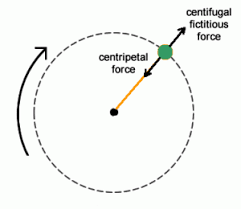The image above represents the centrifugal force.

To compute for the centrifugal force, three essential parameters are needed and these parameters are Mass of the body (m), Angular Velocity of the body (w) and Radius (r).

The formula for calculating the centrifugal force:

F = mω²r

Where:
F = Centrifugal Force
m = mass of the body
ω = angular velocity

Let’s solve an example;
Find the centrifugal force with mass of the body as 12, angular velocity as 32 and a radius of 8.

This implies that;
m = mass of the body = 12
ω = angular velocity = 32

F = mω²r
F = 12 x 32² x 8
F = 12 x 1024 x 8
F = 98304

Therefore, the centrifugal force is 98304 N.

Calculating the Mass of the body (m) when the Centrifugal Force, Angular Velocity and Radius is Given.

m = F / w2r

Where;
m = mass of the body
F = Centrifugal Force
ω = angular velocity

Let’s solve an example;
Find the mass of a body when centrifugal force is 140 with an angular velocity of 24 and a radius of 10.

This implies that;
F = Centrifugal Force = 140
ω = angular velocity = 24

m = F / w2r
m = 140 / 24210
m = 140 / 576 x 10
m = 140 / 5760
m = 0.024

Therefore, the mass of the body is 0.024 kg.

## How to Calculate and Solve the Centre of Gravity of a Segment of a Sphere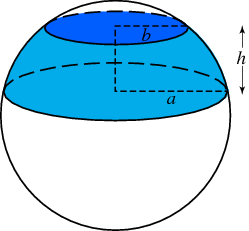The image above represents a segment of a sphere.
To compute the centre of gravity of a segment of a sphere requires two essential parameters. These parameters are the radius of the sphere and height of the segment of the sphere.

The formula for computing the centre of gravity of a sphere is:

C.G. = 3(2r – h)² / 4(3r – h)

Where:
C.G. = Centre of Gravity
r = Radius of the Sphere
h = Height of the Segment of the Sphere

Let’s solve an example
Find the centre of gravity of the segment of the sphere where the radius of the sphere is 10 m and the height of the segment of the sphere is 4 m.

This implies that:
r = Radius of the Sphere = 10
h = Height of the Segment of the Sphere = 4

C.G. = 3(2(10) – 4)² / 4(3(10) – 4)
C.G. = 3(20 – 4)² / 4(30 – 4)
C.G. = 3(16)² / 4(26)
C.G. = 3(256) / 104
C.G. = 768 / 104
C.G. = 7.38

Therefore, the centre of gravity of the segment of the sphere is 7.38.

Nickzom Calculator – The Calculator Encyclopedia is capable of calculating the centre of gravity of a segment of a sphere at a height, h at a distance from the centre of the sphere measured along the height.

## How to Calculate and Solve for the Centre of Gravity of a Cube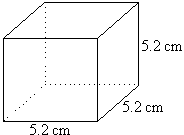The image above is a cube with a length of 5.2 cm.
To compute the centre of gravity of a cube, one essential parameter is needed and this parameter is the length of the cube (l).

The formula for calculating the centre of gravity of a cube is:

C.G. = 0.5(l)

Where:
l = Length of the Cube
C.G. = Centre of Gravity

Let’s solve an example:
Find the centre of gravity of a cube where the length of a side of the cube is 5.2 cm.

This implies that:
l = Length of the Cube = 5.2

## How to Calculate and Solve for the Centroid or Centre of Gravity of a HemisphereThe image above is a hemisphere with a radius of 5.
To compute the centroid or centre of gravity of a hemisphere. You need one essential parameter and this parameter is the radius of the hemisphere (r).

The formula for calculating the centroid or centre of gravity of a hemisphere is:

C.G. = 3r / 8

Where
r = Radius of the hemisphere

As always let us try and solve an example:
Find the centroid or centre of gravity of a hemisphere where the radius is 5 cm.

From the formula this implies that:
r = Radius of the hemisphere = 5

C.G. = 3(5) / 8
C.G. = 15 / 8
C.G. = 1.875

Therefore, the centroid or centre of gravity of the hemisphere is 1.875.

Nickzom Calculator – The Calculator Encyclopedia is capable of calculating the centre of gravity of a hemisphere at a distance from its base measured along the vertical radius.

To get the answer and workings of the center of gravity or centroid of a hemisphere using the Nickzom Calculator – The Calculator Encyclopedia. First, you need to obtain the app.

You can get this app via any of these means:

## Nickzom Calculator Calculates the Centroid or Centre of Gravity of a Semicircle | Statics (Mechanics)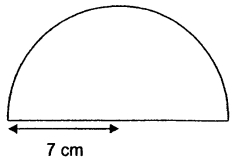The image above is a semicircle with a radius of 7 cm.
To compute the centroid or centre of gravity of a semicircle, you need one essential parameter and this parameter is the radius of the semicircle.

The formula for calculating the centroid or centre of gravity of a semicircle is:

C.G.= 4r /

Where:
r = Radius of the Semicircle
π = Mathematical Constant = 3.142 (approximately)

Let’s solve an example:
Find the centroid or centre of gravity of a semicircle where the radius is 7 cm.

From the example above,
r = radius of the semicircle = 7

C.G. = 4(7) /
C.G. = 28 / 9.4247
C.G. = 2.97

Therefore, the centroid or centre of gravity of the semicircle is 2.97.

Nickzom Calculator – The Calculator Encyclopedia is capable of calculating the centre of gravity of a semicircle at a distance from its base measured along the vertical radius.

To get the answer and workings of the center of gravity or centroid of a semicircle using the Nickzom Calculator – The Calculator Encyclopedia. First, you need to obtain the app.

## How to Calculate and Solve for the Centroid or Centre of Gravity of a Sphere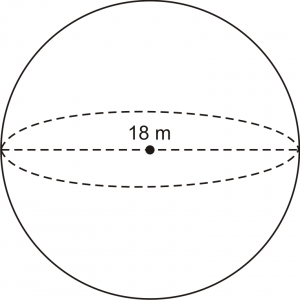The image above is a sphere and 18 m is the diameter of the sphere. In the computing of the centroid or centre of gravity of a sphere there is only one essential parameter which is the diameter of the sphere.

The formula for calculating the centroid or centre of gravity of a sphere is:

C.G. = d / 2

Where:

d is the diameter of the sphere

As always, let’s take an example:

Let’s solve an example
Find the centroid or centre of gravity of a sphere where the diameter is 18 m.

C.G. = 18 / 2
C.G. = 9

Therefore, the centroid or centre of gravity of the sphere is 9.

Nickzom Calculator – The Calculator Encyclopedia is capable of calculating the centre of gravity of a sphere at a distance from every point.

To get the answer and workings of the center of gravity or centroid of a sphere using the Nickzom Calculator – The Calculator Encyclopedia. First, you need to obtain the app.

You can get this app via any of these means:

## How to Calculate and Solve the Centre of Gravity of a Right Circular Cone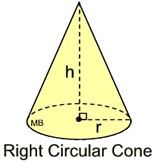This image above is a display of what a right circular cone looks like. There is only one essential parameter for calculating the centroid or centre of gravity of a right circular cone. This parameter is the height of the cone (h).

The formula for calculating the the centroid or centre of gravity of a right circular cone is:

C.G. = h / 4

As always let’s solve an example.

Find the centroid or centre of gravity of a right circular cone where the height of the cone is 12cm.
This implies that:

h = height of the cone = 12

C.G. = 12 / 4
C.G. = 3

Therefore, the centroid or centre of gravity of the right circular cone is 3.

Nickzom Calculator – The Calculator Encyclopedia is capable of calculating the centre of gravity of a right circular cone at a distance from its base measured along the vertical axis.

To get the answer and workings to center of gravity or centroid of a right circular cone using the Nickzom Calculator – The Calculator Encyclopedia. First, you need to obtain the app.

You can get this app via any of these means:

## How to Calculate the Centre of Gravity of a Circular Sector in Statics | Mechanics

It is very possible to compute the centroid or centre of gravity of a circular sector. There are two highly important parameters one needs to know to compute the centre of gravity of a circular sector. These parameters are:

• Radius of the Sector (r)
• Semi Vertical Angle (α)

The formula for computing the centre of gravity of a circular sector is:

C.G. = 2rsinα /

Now, let’s take an example.
Let’s find the centroid or the centre of gravity of a circular sector that has a radius of 4m and a semi vertical angle of 30°.
This implies that:

r = Radius of the Sector = 4
α = Semi Vertical Angle = 30

Entering this values into the formula we have:

C.G. = 2(4)sin30° / 3(30)
C.G. = 8 . sin30° / 90
C.G. = 8 . (0.5) / 90
C.G. = 4 / 90
C.G. = 0.0444

Therefore, the centroid or centre of gravity of the circular sector is 0.0444.

Nickzom Calculator – The Calculator Encyclopedia is capable of calculating the centre of gravity of a circular sector making a semi vertical angle α at a distance from the centre of the sector measured along the central axis.

To get the answer and workings to center of gravity or centroid of a circular sector. First, you need to obtain the Nickzom Calculator – The Calculator Encyclopedia app.

## Nickzom Calculator Calculates the Center of Gravity of a Trapezium in Statics Mechanics

According to Quora,

The center of gravity of a trapezoid can be estimated by dividing the trapezoid in two triangles.

Nickzom Calculator calculates the centre of gravity of a trapezium with parallel sides a and b at a distance measured from side b.

The formula for calculating this center of gravity is:

C.G. = h (b + 2a) / 3 (b + a)

Where, a and b are the length of the parallel sides of the trapezium (b being the base length and a being the top length) whereas h is the height of the trapezium.

Let’s take for Example: Find the center of gravity or centroid of a trapezium where a is 4, b is 8 and h is 2.

This implies:

a = 4
b = 8
h = 2

C.G. = 2 (8 + 2(4)) / 3 (8 + 4)
C.G. = 2 (8 + 8) / 3 (12)
C.G. = 2 (16) / 36
C.G. = 32 / 36
C.G. = 0.89

Therefore, the center of gravity or centroid of the trapezium is 0.89.

To get the answer and workings to center of gravity or centroid of a trapezium. First, you need to obtain the Nickzom Calculator – The Calculator Encyclopedia app.